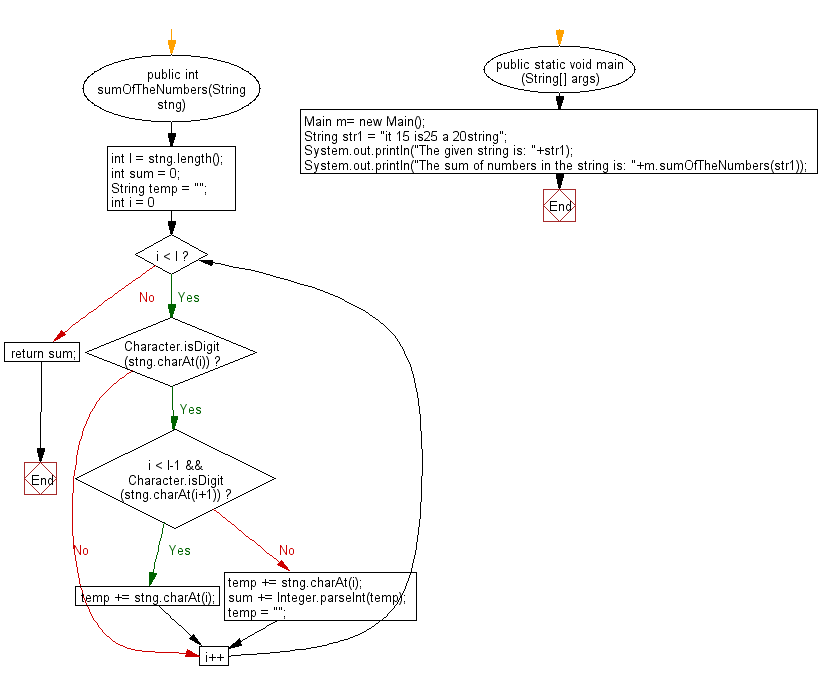﻿ Java exercises: Calculate the sum of the numbers appear in a given string - w3resource# Java String Exercises: Calculate the sum of the numbers appear in a given string

## Java String: Exercise-89 with Solution

Write a Java program to calculate the sum of the numbers appear in a given string.

Sample Solution:

Java Code:

``````import java.util.*;
public class Main
{
public int sumOfTheNumbers(String stng)
{
int l = stng.length();
int sum = 0;
String temp = "";
for (int i = 0; i < l; i++)
{
if (Character.isDigit(stng.charAt(i)))
{
if (i < l-1 && Character.isDigit(stng.charAt(i+1)))
{
temp += stng.charAt(i);
}
else
{
temp += stng.charAt(i);
sum += Integer.parseInt(temp);
temp = "";
}
}
}
return sum;
}

public static void main (String[] args)
{
Main m= new Main();
String str1 =  "it 15 is25 a 20string";
System.out.println("The given string is: "+str1);
System.out.println("The sum of numbers in the string is: "+m.sumOfTheNumbers(str1));
}
}
``````

Sample Output:

```The given string is: it 15 is25 a 20string
The sum of numbers in the string is: 60
```

Pictorial Presentation:Flowchart:Java Code Editor:

Improve this sample solution and post your code through Disqus

What is the difficulty level of this exercise?

﻿

## Java: Tips of the Day

Array vs ArrayLists:

The main difference between these two is that an Array is of fixed size so once you have created an Array you cannot change it but the ArrayList is not of fixed size. You can create instances of ArrayLists without specifying its size. So if you create such instances of an ArrayList without specifying its size Java will create an instance of an ArrayList of default size.

Once an ArrayList is full it re-sizes itself. In fact, an ArrayList is internally supported by an array. So when an ArrayList is resized it will slow down its performance a bit as the contents of the old Array must be copied to a new Array.

At the same time, it's compulsory to specify the size of an Array directly or indirectly while creating it. And also Arrays can store both primitives and objects while ArrayLists only can store objects.

Ref: https://bit.ly/3o8L2KH